# layout

Change layout of graph plot

## Syntax

``layout(H)``
``layout(H,method)``
``layout(H,method,Name,Value)``

## Description

example

````layout(H)` changes the layout of graph plot `H` by using an automatic choice of layout method based on the structure of the graph. The `layout` function modifies the `XData` and `YData` properties of `H`.```

example

````layout(H,method)` optionally specifies the layout method. `method` can be `'circle'`, `'force'`, `'layered'`, `'subspace'`, `'force3'`, or `'subspace3'`.```

example

````layout(H,method,Name,Value)` uses additional options specified by one or more name-value pair arguments. For example, `layout(H,'force','Iterations',N)` specifies the number of iterations to use in computing the force layout, and `layout(H,'layered','Sources',S)` uses a layered layout with source nodes `S` included in the first layer.```

## Examples

collapse all

Create and plot a graph using the `'force'` layout.

```s = [1 1 1 1 1 6 6 6 6 6]; t = [2 3 4 5 6 7 8 9 10 11]; G = graph(s,t); h = plot(G,'Layout','force');```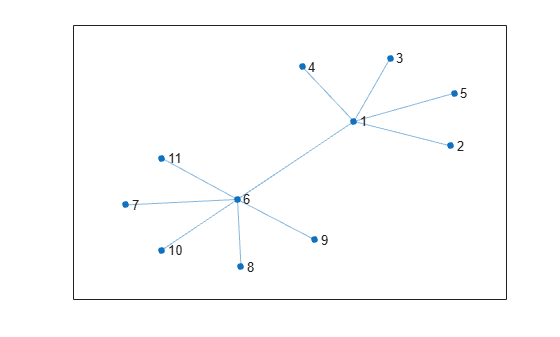Change the layout to be the default that `plot` determines based on the structure and properties of the graph. The result is the same as using `plot(G)`.

`layout(h)`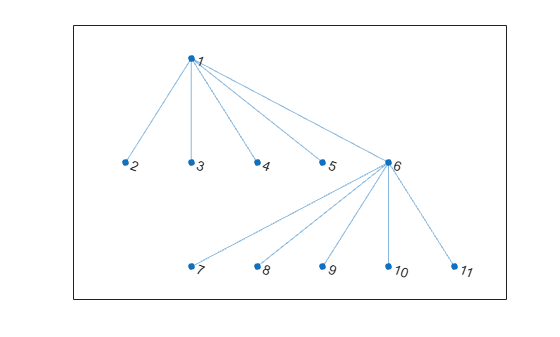Create and plot a graph using the `'layered'` layout.

```s = [1 1 1 2 2 3 3 4 5 5 6 7]; t = [2 4 5 3 6 4 7 8 6 8 7 8]; G = graph(s,t); h = plot(G,'Layout','layered');```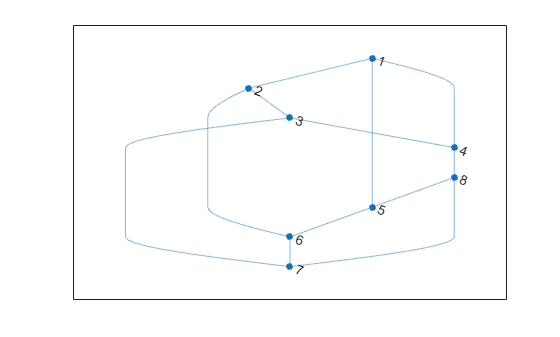Change the layout of the graph to use the `'subspace'` method.

`layout(h,'subspace')`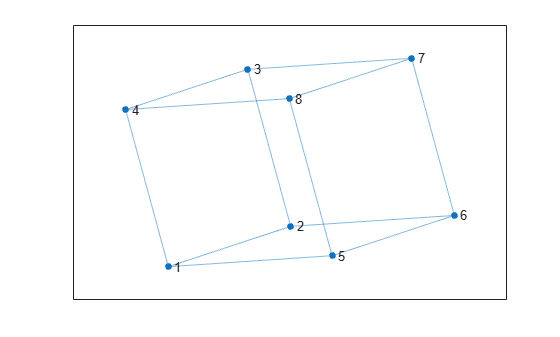Create and plot a graph using the `'layered'` layout method.

```s = [1 1 1 2 3 3 3 4 4]; t = [2 4 5 6 2 4 7 8 1]; G = digraph(s,t); h = plot(G,'Layout','layered');```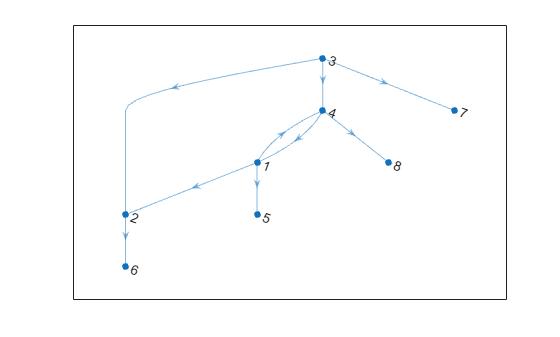Use the `layout` function to refine the hierarchical layout by specifying source nodes and a horizontal orientation.

`layout(h,'layered','Direction','right','Sources',[1 4])`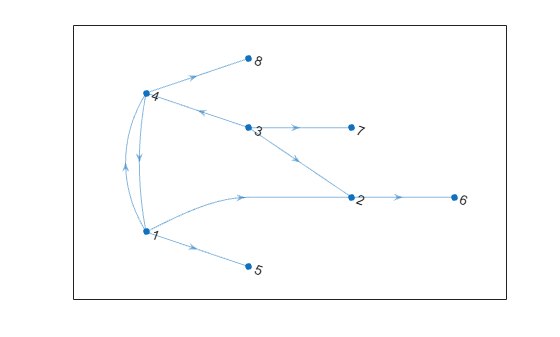Plot a graph that has multiple components, and then show how to use the `'UseGravity'` option to improve the visualization.

Create and plot a graph that has 150 nodes separated into many disconnected components. MATLAB® lays the graph components out on a grid.

```s = [1 3 5 7 7 10:100]; t = [2 4 6 8 9 randi([10 100],1,91)]; G = graph(s,t,[],150); h = plot(G);```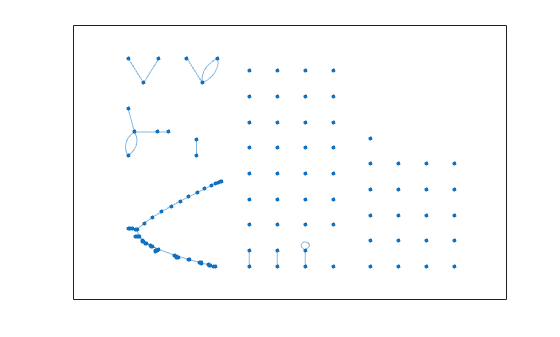Update the layout coordinates of the graph object, and specify `'UseGravity'` as `true` so that the components are layed out radially around the origin, with more space allotted for the larger components.

`layout(h,'force','UseGravity',true)`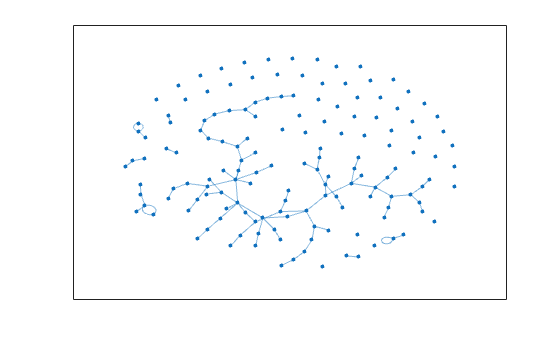Plot a graph using the `'WeightEffect'` name-value pair to make the length of graph edges proportional to their weights.

Create and plot a directed graph with weighted edges.

```s = [1 1 1 1 1 2 2 2 3 3 3 3 3]; t = [2 4 5 6 7 3 8 9 10 11 12 13 14]; weights = randi([1 20],1,13); G = graph(s,t,weights); p = plot(G,'Layout','force','EdgeLabel',G.Edges.Weight);```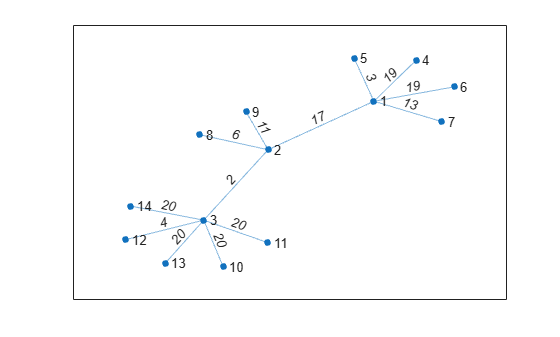Recompute the layout of the graph using the `'WeightEffect'` name-value pair, so that the length of each edge is proportional to its weight. This makes it so that the edges with the largest weights are the longest.

`layout(p,'force','WeightEffect','direct')`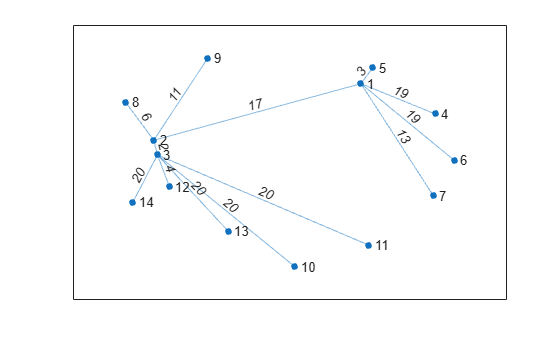## Input Arguments

collapse all

Input graph plot, specified as a `GraphPlot` object. Use the `graph` or `digraph` functions to create a graph, and then use `plot` with an output argument to return a `GraphPlot` object.

Example: `H = plot(G)`

Layout method, specified as one of the options in the table. The table also lists compatible name-value pairs to further refine each layout method.

OptionDescriptionLayout-Specific Name-Value Pairs
`'auto'` (default)

Automatic choice of layout method based on the size and structure of the graph.

`'circle'`

Circular layout. Places the graph nodes on a circle centered at the origin with radius 1.

`'Center'` — Center node in circular layout

`'force'`

Force-directed layout . Uses attractive forces between adjacent nodes and repulsive forces between distant nodes.

`'Iterations'` — Number of force-directed layout iterations

`'WeightEffect'` — Effect of edge weights on layout

`'UseGravity'` — Gravity toggle for layouts with multiple components

`'XStart'` — Starting x-coordinates for nodes

`'YStart'` — Starting y-coordinates for nodes

`'layered'`

Layered layout , , . Places the graph nodes into a set of layers, revealing hierarchical structure. By default the layers progress downwards (the arrows of a directed acyclic graph point down).

`'Direction'` — Direction of layers

`'Sources'` — Nodes to include in the first layer

`'Sinks'` — Nodes to include in the last layer

`'AssignLayers'` — Layer assignment method

`'subspace'`

Subspace embedding layout . Plots the graph nodes in a high-dimensional embedded subspace, and then projects the positions back into 2-D. By default the subspace dimension is either 100 or the total number of nodes, whichever is smaller.

`'Dimension'` — Dimension of embedded subspace

`'force3'`3-D force-directed layout.

`'Iterations'` — Number of force-directed layout iterations

`'WeightEffect'` — Effect of edge weights on layout

`'UseGravity'` — Gravity toggle for layouts with multiple components

`'XStart'` — Starting x-coordinates for nodes

`'YStart'` — Starting y-coordinates for nodes

`'ZStart'` — Starting z-coordinates for nodes

`'subspace3'`3-D subspace embedding layout.

`'Dimension'` — Dimension of embedded subspace

Example: `layout(H,'layered')`

Example: `layout(H,'force3','Iterations',10)`

Example: `layout(H,'subspace','Dimension',50)`

### Name-Value Pair Arguments

Specify optional comma-separated pairs of `Name,Value` arguments. `Name` is the argument name and `Value` is the corresponding value. `Name` must appear inside quotes. You can specify several name and value pair arguments in any order as `Name1,Value1,...,NameN,ValueN`.

Example: `layout(H,'subspace','Dimension',200)`
Force

collapse all

Number of force-directed layout iterations, specified as the comma-separated pair consisting of `'Iterations'` and a positive scalar integer.

This option is available only when `method` is `'force'` or `'force3'`.

Example: `layout(H,'force','Iterations',250)`

Data Types: `single` | `double` | `int8` | `int16` | `int32` | `int64` | `uint8` | `uint16` | `uint32` | `uint64`

Effect of edge weights on layout, specified as the comma-separated pair consisting of `'WeightEffect'` and one of the values in this table. If there are multiple edges between two nodes (as in a directed graph with an edge in each direction, or a multigraph), then the weights are summed before computing `'WeightEffect'`.

This option is available only when `method` is `'force'` or `'force3'`.

Value

Description

`'none'` (default)

Edge weights do not affect the layout.

`'direct'`

Edge length is proportional to the edge weight, `G.Edges.Weight`. Larger edge weights produce longer edges.

`'inverse'`

Edge length is inversely proportional to the edge weight, `1./G.Edges.Weight`. Larger edge weights produce shorter edges.

Example: `layout(H,'force','WeightEffect','inverse')`

Gravity toggle for layouts with multiple components, specified as the comma-separated pair consisting of `'UseGravity'` and either `'off'`, `'on'`, `true`, or `false`. A value of `'on'` is equivalent to `true`, and `'off'` is equivalent to `false`.

By default, MATLAB® lays out graphs with multiple components on a grid. The grid can obscure the details of larger components since they are given the same amount of space as smaller components. With `'UseGravity'` set to `'on'` or `true`, multiple components are instead layed out radially around the origin. This layout spreads out the components in a more natural way, and provides more space for larger components.

This option is available only when `method` is `'force'` or `'force3'`.

Example: `layout(H,'force','UseGravity',true)`

Data Types: `char` | `logical`

Starting x-coordinates for nodes, specified as the comma-separated pair consisting of `'XStart'` and a vector of node coordinates. Use this option together with `'YStart'` to specify 2-D starting coordinates (or with `'YStart'` and `'ZStart'` to specify 3-D starting coordinates) before iterations of the force-directed algorithm change the node positions.

This option is available only when `method` is `'force'` or `'force3'`.

Example: `layout(H,'force','XStart',x,'YStart',y)`

Data Types: `single` | `double` | `int8` | `int16` | `int32` | `int64` | `uint8` | `uint16` | `uint32` | `uint64`

Starting y-coordinates for nodes, specified as the comma-separated pair consisting of `'YStart'` and a vector of node coordinates. Use this option together with `'XStart'` to specify 2-D starting coordinates (or with `'XStart'` and `'ZStart'` to specify 3-D starting coordinates) before iterations of the force-directed algorithm change the node positions.

This option is available only when `method` is `'force'` or `'force3'`.

Example: `layout(H,'force','XStart',x,'YStart',y)`

Example: `layout(H,'force','XStart',x,'YStart',y,'ZStart',z)`

Data Types: `single` | `double` | `int8` | `int16` | `int32` | `int64` | `uint8` | `uint16` | `uint32` | `uint64`

Starting z-coordinates for nodes, specified as the comma-separated pair consisting of `'ZStart'` and a vector of node coordinates. Use this option together with `'XStart'` and `'YStart'` to specify the starting x, y, and z node coordinates before iterations of the force-directed algorithm change the node positions.

This option is available only when `method` is `'force3'`.

Example: `layout(H,'force','XStart',x,'YStart',y,'ZStart',z)`

Data Types: `single` | `double` | `int8` | `int16` | `int32` | `int64` | `uint8` | `uint16` | `uint32` | `uint64`

Layered

collapse all

Direction of layers, specified as the comma-separated pair consisting of `'Direction'` and either `'down'`, `'up'`, `'left'` or `'right'`. For directed acyclic (DAG) graphs, the arrows point in the indicated direction.

This option is available only when `method` is `'layered'`.

Example: `layout(H,'layered','Direction','up')`

Nodes to include in the first layer, specified as the comma-separated pair consisting of `'Sources'` and one or more node indices or node names.

This table shows the different ways to refer to one or more nodes either by their numeric node indices or by their node names.

FormSingle NodeMultiple Nodes
Node index

Scalar

Example: `1`

Vector

Example: `[1 2 3]`

Node name

Character vector

Example: `'A'`

Cell array of character vectors

Example: `{'A' 'B' 'C'}`

String scalar

Example: `"A"`

String array

Example: `["A" "B" "C"]`

This option is available only when `method` is `'layered'`.

Example: ```layout(H,'layered','Sources',[1 3 5])```

Nodes to include in the last layer, specified as the comma-separated pair consisting of `'Sinks'` and one or more node indices or node names.

This option is available only when `method` is `'layered'`.

Example: ```layout(H,'layered','Sinks',[2 4 6])```

Layer assignment method, specified as the comma-separated pair consisting of `'AssignLayers'` and one of the options in this table.

OptionDescription
`'auto'` (default)Node assignment uses either `'asap'` or `'alap'`, whichever is more compact.
`'asap'`As soon as possible. Each node is assigned to the first possible layer, given the constraint that all its predecessors must be in earlier layers.
`'alap'`As late as possible. Each node is assigned to the last possible layer, given the constraint that all its successors must be in later layers.

This option is available only when `method` is `'layered'`.

Example: `layout(H,'layered','AssignLayers','alap')`

Subspace

collapse all

Dimension of embedded subspace, specified as the comma-separated pair consisting of `'Dimension'` and a positive scalar integer.

• The default integer value is ```min([100, numnodes(G)])```.

• For the `'subspace'` layout, the integer must be greater than or equal to 2.

• For the `'subspace3'` layout, the integer must be greater than or equal to 3.

• In both cases, the integer must be less than the number of nodes.

This option is available only when `method` is `'subspace'` or `'subspace3'`.

Example: `layout(H,'subspace','Dimension',d)`

Data Types: `single` | `double` | `int8` | `int16` | `int32` | `int64` | `uint8` | `uint16` | `uint32` | `uint64`

Circle

collapse all

Center node in circular layout, specified as the comma-separated pair consisting of `'Center'` and one of the values in this table.

ValueExample
Scalar node index`1`
Character vector node name`'A'`
String scalar node name`"A"`

This option is available only when `method` is `'circle'`.

Example: `layout(H,'circle','Center',3)` places node three at the center.

Example: `layout(H,'circle','Center','Node1')` places the node named `'Node1'` at the center.

## Tips

• Use the `Layout` name-value pair to change the layout of a graph when you plot it. For example, `plot(G,'Layout','circle')` plots the graph `G` with a circular layout.

• When using the `'force'` or `'force3'` layout methods, a best practice is to use more iterations with the algorithm instead of using `XStart`, `YStart`, and `ZStart` to restart the algorithm using previous outputs. The result of executing the algorithm with 100 iterations is different in comparison to executing 50 iterations, and then restarting the algorithm from the ending positions to execute 50 more iterations.

 Fruchterman, T., and E. Reingold,. “Graph Drawing by Force-directed Placement.” Software — Practice & Experience. Vol. 21 (11), 1991, pp. 1129–1164.

 Gansner, E., E. Koutsofios, S. North, and K.-P Vo. “A Technique for Drawing Directed Graphs.” IEEE Transactions on Software Engineering. Vol.19, 1993, pp. 214–230.

 Barth, W., M. Juenger, and P. Mutzel. “Simple and Efficient Bilayer Cross Counting.” Journal of Graph Algorithms and Applications. Vol.8 (2), 2004, pp. 179–194.

 Brandes, U., and B. Koepf. “Fast and Simple Horizontal Coordinate Assignment.” LNCS. Vol. 2265, 2002, pp. 31–44.

 Y. Koren. “Drawing Graphs by Eigenvectors: Theory and Practice.” Computers and Mathematics with Applications. Vol. 49, 2005, pp. 1867–1888.